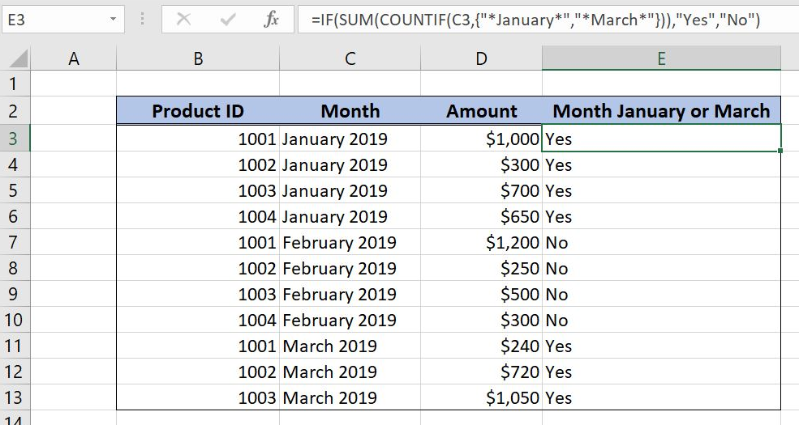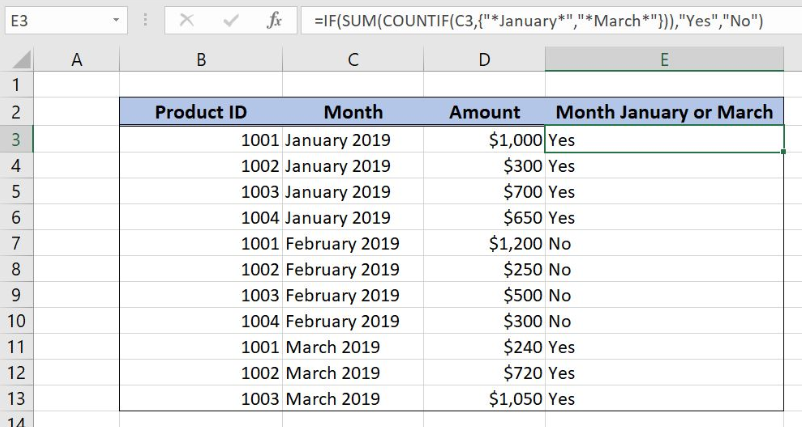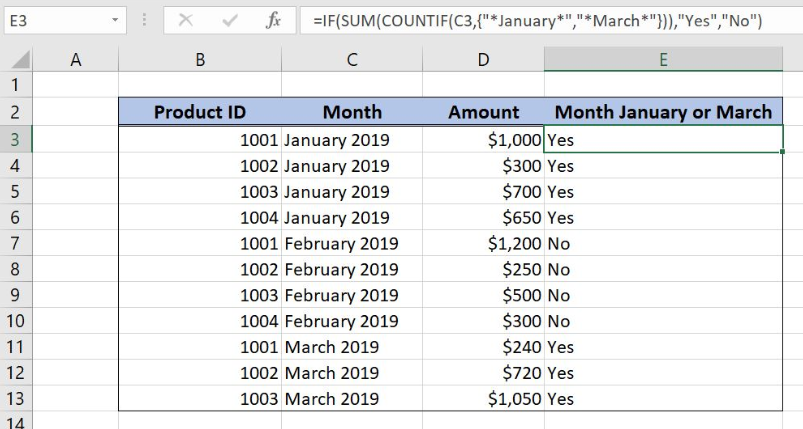Get instant live expert help with Excel or Google Sheets“My Excelchat expert helped me in less than 20 minutes, saving me what would have been 5 hours of work!”

#### Post your problem and you’ll get Expert help in seconds.

Your message must be at least 40 characters
Our professional Expert are available now. Your privacy is guaranteed.

# If cell contains this or that

Excel allows a user to check if a range of cells contains any of two selected values using the COUNTIF, SUM and IF functions. This step by step tutorial will assist all levels of Excel users in checking if cells contain this or that.Figure 1. The result of the formula

## Syntax of the COUNTIF Formula

The generic formula for the COUNTIF function is:

`=COUNTIF(range, criteria)`

The parameters of the COUNTIF function are:

• range – a range where we want to apply our criteria
• criteria – a criteria in a range which we want to count.

## Syntax of the SUM Formula

The generic formula for the SUM function is:

`=SUM(number1, number2, …, numberN)`

The parameters of the SUM function are:

• number1, number2, …, numberN – numbers which we want to sum.

## Syntax of the IF Formula

The generic formula for the IF function is:

`=IF(logical_test, value_if_true, value_if_false)`

The parameters of the IF function are:

• logical_test – a logical expression that we want to check
• value_if_true – a value which the function returns if a logical_test is TRUE
• value_if_false – a value which the function returns if a logical_test is FALSE.

## Setting up Our Data for the Formula

In the data table, we have 4 columns: “Product ID” (column B), “Month” (column C), “Amount” (column D) and “Month January or March” (column E). In column E, we want to check if a value in “Month” column contains January or March. If this is true, the result will be “Yes”, otherwise “No”.Figure 2. Data that we will use in the example

## Checking if Cells Contain Any of Two Values

In our example, we want to check if the value in C3 contains January or March. If this is true, the result in E3 will be “Yes” and if it’s not “No”.

The formula looks like:

`=IF(SUM(COUNTIF(C3, {"*January*", "*March*"})), "Yes", "No")`

The parameter range is the cell C3 and the criteria is the array {“January”, “March”}. The result of this COUNTIF functions are numbers (zero, one or two numbers), which are number parameters of the SUM function. Finally, the result of the SUM function is the logical_test for the IF function. Value_if_true is “Yes” and Value_if_false is “No”.

To apply the formula, we need to follow these steps:

• Select cell E3 and click on it
• Insert the formula: `=IF(SUM(COUNTIF(C3,{"*January*","*March*"})),"Yes","No")`
• Press enter
• Drag the formula down to the other cells in the column by clicking and dragging the little “+” icon at the bottom-right of the cell.Figure 3. Counting items with multiple criteria and or logic

As you can see, the cell C3 contains “January”, so the COUNTIF function returns 1 and the SUM also. As this number is different than 0, the IF logical condition is true, so the function returns value_if_true. Therefore, the result in the cell E3 is “Yes”.

Most of the time, the problem you will need to solve will be more complex than a simple application of a formula or function. If you want to save hours of research and frustration, try our live Excelchat service! Our Excel Experts are available 24/7 to answer any Excel question you may have. We guarantee a connection within 30 seconds and a customized solution within 20 minutes.

Are you still looking for help with the IF function? View our comprehensive round-up of IF function tutorials here.

### Did this post not answer your question? Get a solution from connecting with the expert.Another blog reader asked this question today on Excelchat:
Solution examplesI am trying to have a tab change color when the Sum of a row = 0. I am using code on the tab but it appears to be confused because the value of the given cell is not 0 it is "=SUM(G4:G17)"
Solved by S. F. in 41 minsCould you help me by explaining the IF function?
Solved by G. L. in 24 minsI have a question about Conditional Formatting. =IF('Sheet1'!A1="X",IF('Sheet1'!B1="Y", TRUE, FALSE),FALSE) the set the cell background to red. I would like to make it so that if Sheet1'!B1="Z" I set the back to blue. How can I achieve this.
Solved by B. F. in 39 minsI need to find out if the 2018 yearly sales goals were met if the yearly sales were \$25,000 or more using an IF logical function and to set the formula to return a value of YES if met, and NO if not
Solved by A. A. in 18 minsI filtered data in column A (it is labeled ID #) of a data set. On my next worksheet, column A is also the ID #, but it is a different data set. I want to filter the ID #'s the same for the two sheets, but how do I do that being that I am working with two different sets of data?
Solved by V. H. in 22 mins## Subscribe to Excelchat.coAnother blog reader asked this question today on Excelchat: